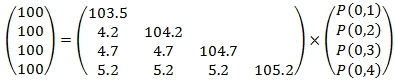# SQL Server Constant Maturity Treasury curve

CMTCurve
Updated: 04 Jan 2017
Use the table-valued function CMTCurve to generate a yield curve using Constant Maturity Treasury (CMT) rates or other similar rate types.
CMTCurve expects the rates to be supplied using dynamic SQL in which the resultant table consists of the time (in years) and the rate (where 10% = 0.10).
CMTCurve supports annual (1) and semi-annual (2) compounding. It assumes that all supplied rates which have a time value less than or equal to 1 / compounding frequency are cash rates which can be directly converted into discount factors. For all other rates, a bootstrapping processing is used to calculate the discount factors. Bootstrapped rates are identified as such in the table returned by the function. CMTCurve supports linear and spline interpolation for all the coupons. If we were to generate a lower triangular matrix containing the (calculated) cash flows for each point on the yield curve, then the calculation of the discount factors is straightforward forward substitution for each of the interpolated points.
For example, given the following annual rates:

 T R 1 .035 2 .042 3 .047 4 .052

We are able to directly calculate the discount factors for each point by setting up the following equation:Which can be solved directly in SQL Server using the existing XLeratorDB math functions Matrix2String_q, MUPDATE, EYE, FWDSUB, MTRIL, and Matrix.
--Put the par rates into matrix format
DECLARE @cf as varchar(max) = wct.Matrix2String_q('
SELECT r
FROM (VALUES (1,.035),(2,.042),(3,.047),(4,.052))n(T,r)
ORDER BY T'
)

--Generate the cash flows in matrix format
SET @cf = wct.MUPDATE(100,NULL,NULL,NULL,NULL,'*',wct.MUPDATE(@cf,NULL,NULL,NULL,NULL,'+',wct.EYE(4,4),NULL,NULL,NULL,NULL),NULL,NULL,NULL,NULL)

--Solve by forward substitution
SELECT
rownum + 1 as T
,ItemValue as df
FROM
wct.Matrix(wct.FWDSUB(wct.MTRIL(@cf),'100;100;100;100'))
This produces the following result.
CMTCurve simplifies the SQL into a single, easy-to-use function call.
Syntax
SELECT * FROM [wct].[CMTCurve](
<@Curve, nvarchar(max),>
,<@InterpMethod, nvarchar(4000),>
,<@Freq, int,>)
Arguments
@Curve
A SELECT statement which return a resultant consisting of the time (in years) and the associated rates (where 10% = 0.10). @Curve should return 2 columns.
@InterpMethod
Identifies the interpolation method used in the bootstrapping process. Use 'S' for spline interpolation, 'L0' for linear interpolation where bounds are used for any values that would otherwise be out-of-bonds, or 'L1' for linear interpolation with extrapolation.
@Freq
Use 1 for annual or 2 for semi-annual. @Freq is an expression of type int or of a type that can be implicitly converted to int.
Return Type
RETURNS TABLE(
[T] [float] NULL,
[r] [float] NULL,
[df] [float] NULL,
[spot] [float] NULL,
[cczero] [float] NULL,
[bootstrap] [bit] NULL
)

 Column Description T Time (in years) associated with the returned rate r Par rate df Discount factor spot Spot rate; @Freq*(POWER(1/df,1/(@Freq*T))-1 cczero Continuously compounded zero rate; -LOG(df)/T bootstrap identifies T and r as having come from @Curve (1) or having been interpolated (0)

Remarks
·         If @Curve returns less than 2 columns an error is generated
·         NULL values returned by @Curve are discarded
·         Only 1 r value should be returned for each T in @Curve
·         If @InterpMethod is NULL then @InterpMethod = 'S'
·         If @Freq is NULL then @Freq = 2
·         Available in XLeratorDB / financial 2008 only
Examples
Example #1
Using the data from the introduction.
SELECT
*
FROM
wct.CMTCurve('
SELECT T, r
FROM (VALUES (1, .035), (2, .042), (3, .047), (4, .052))n(T, r)
ORDER BY T'   --@Curve
,'L'          --@InterpMethod
,1            --@Freq
)
This produces the following result.
Example #2
In this example we put the curve into a temp table, #cmt, and have the dynamic SQL select from #cmt.
SELECT
T
,r/100 as R
INTO
#cmt
FROM (VALUES
(0.0833333333333333,0.44)
,(0.25,0.51)
,(0.5,0.62)
,(1,0.85)
,(2,1.2)
,(3,1.47)
,(5,1.93)
,(7,2.25)
,(10,2.45)
,(20,2.79)
,(30,3.06)
)n(T,r)

SELECT
*
FROM
wct.CMTCurve('
SELECT T,R
FROM #cmt' --@Curve
,NULL     --@InterpMethod
,NULL     --@Freq
)
This produces the following result.
Example #3
Using the same data from Example #2, but this time only selecting the rows where bootstrap is false.
SELECT
T
,r/100 as R
INTO
#cmt
FROM (VALUES
(0.0833333333333333,0.44)
,(0.25,0.51)
,(0.5,0.62)
,(1,0.85)
,(2,1.2)
,(3,1.47)
,(5,1.93)
,(7,2.25)
,(10,2.45)
,(20,2.79)
,(30,3.06)
)n(T,r)

SELECT
*
FROM
wct.CMTCurve('
SELECT T,R
FROM #cmt' --@Curve
,NULL     --@InterpMethod
,NULL     --@Freq
)
WHERE
bootstrap = 'False'
This produces the following result.
Example #4
In this example we use the same data as in the previous 2 example, but no we are going to shift the par curve up and down by 25 basis points and then return the continuously compounded zeroes for par rates and the shifted rates.
SELECT
T
,r/100 as R
INTO
#cmt
FROM (VALUES
(0.0833333333333333,0.44)
,(0.25,0.51)
,(0.5,0.62)
,(1,0.85)
,(2,1.2)
,(3,1.47)
,(5,1.93)
,(7,2.25)
,(10,2.45)
,(20,2.79)
,(30,3.06)
)n(T,r)

SELECT
x.Ztype
,k.T
,k.cczero
INTO
#z
FROM (VALUES
('Z', 'SELECT T,r FROM #cmt')
,('Zplus','SELECT T,r+.0025 FROM #cmt')
,('Zminus','SELECT T,r-.0025 FROM #cmt')
)x(Ztype,ZSQL)
CROSS APPLY
wct.CMTCURVE(x.ZSQL,'S',2)k
WHERE
bootstrap = 'False'

SELECT T, Z, zPlus, Zminus
FROM (SELECT * FROM #z) pvt
PIVOT(MAX(cczero) for Ztype in(Z,Zplus,Zminus))d
This produces the following result.

### SupportCopyright 2008-2021 Westclintech LLC         Privacy Policy        Terms of Service